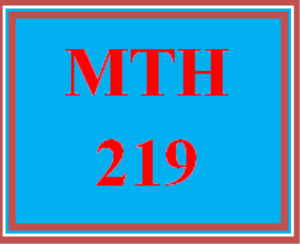# MTH 219T Wk 3 – Midterm Exam

PLDZ-13173 Free In Stock
\$ 0.00 USD
Description

***************************************************************

~~~~~~~~~~~~~~~~~~~~~~~~~~~~~~~~~~~~~~~~~~~~~~~~~

https://uopcourse.com/

***************************************************************

MTH 219T Wk 3 – Midterm Exam

Complete the midterm exam. You have one attempt at the exam. So, be sure to review all previous course materials before attempting the exam.

Question 1

Suppose x is the height of a building and y is the number of rooms in the building. Which quadrant does the point (x,y) lie on?

Question 2

If left parenthesis 3 to the power of 8 right parenthesis to the power of 4 equals 3 to the power of x , find x.

2

4

12

32

Question 3

Solve 1 third left parenthesis 3 x plus 6 right parenthesis plus 2 x equals 26 .

x equals 28 over 3

x equals 32 over 5

x equals 4

x equals 8

Question 4

Find the y-intercept of the line fraction numerator 6 y plus 2 x over denominator 5 end fraction equals 18 .

45

-15

-45

15

Question 5

Simplify fraction numerator left parenthesis x to the power of negative 3 end exponent x to the power of 5 right parenthesis left parenthesis 3 y right parenthesis cubed over denominator x to the power of negative 1 end exponent x squared end fraction .

9 x y cubed

9 x cubed y

27 x y cubed

27 x squared y cubed

Question 6

Which of the following is a polynomial?

x cubed plus x y minus 1

begin mathsize 10px style 1 over x end style

x to the power of 1 divided by 2 end exponent 12 y minus 1

fraction numerator x cubed over denominator x minus 1 end fraction

Question 7

Simplify left parenthesis x minus y minus 5 right parenthesis left parenthesis x minus 2 right parenthesis .

2 x minus y minus 3

x squared minus x y plus 7 x plus 2 y plus 10

2 x minus y minus 7

x squared minus x y minus 7 x plus 2 y plus 10

Question 8

Find the value of f left parenthesis 6 right parenthesis space i f space f left parenthesis x right parenthesis equals 1 over 6 x plus 7 .

Recent Reviews Write a Review
0 0 0 0 reviews